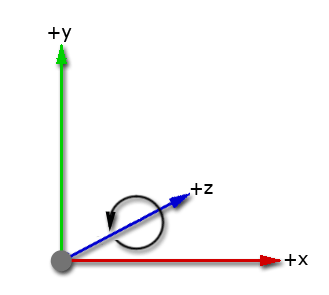Version: 2022.3
Language : English
Scale Constraints
Lights

# Rotation and orientation in Unity

Unity uses a left-handed coordinate system. In Unity, you can use both Euler angles and quaternions to represent rotations and orientation. These representations are equivalent but have different uses and limitations.

Typically, you rotate objects in your using the , which displays orientation as a Euler angle. However, Unity stores rotations and orientations internally as quaternions, which can be useful for more complex motions that might otherwise lead to gimbal lock.

## Left-handed coordinate systemThe axes of Unity’s left-handed coordinate system, showing that the direction of rotation from the positive x-axis to the positive y-axis is counterclockwise when looking along the positive z-axis.

A coordinate system describes the position of objects in a three-dimensional space. Unity uses a left-handed coordinate system: the positive x-axis points to the right, the positive y-axis points up, and the positive z-axis points forward. Unity’s left-handed coordinate system means that the direction of rotation from the positive x-axis to the positive y-axis is counterclockwise when looking along the positive z-axis.

## Euler angles

In the Transform coordinate, Unity displays rotation with the vector property `Transform.eulerAngles` X, Y, and Z. Unlike a normal vector, these values actually represent the angle (in degrees) of rotation about the X, Y, and Z axes.

Euler angle rotations perform three separate rotations around the three axes. Unity performs the Euler rotations sequentially around the z-axis, the x-axis and then the y-axis. This method of rotation is extrinsic rotation; the original coordinate system doesn’t change while the rotations occur.

To rotate a , you can enter angle values of how far you want each axis to rotate into the Transform component. To rotate your GameObjects with script, use `Transform.eulerAngles`. If you convert to Euler angles to do calculations and rotations, you risk problems with gimbal lock.

### Gimbal lock

When an object in 3D space loses a degree of freedom and can only rotate within two dimensions, it’s called gimbal lock. Gimbal lock can occur with Euler angles if two axes become parallel. If you don’t convert the rotational values to Euler angles in your script, the use of quaternions should prevent gimbal lock.

If you do have problems with gimbal lock, you can avoid Euler angles if you use `Transform.RotateAround` for rotations. You can also use `Quaternion.AngleAxis` on each axis and multiply them together (quaternion multiplication applies each rotation in turn).

## Quaternions

Quaternions provide mathematical notation for unique representations of spatial orientation and rotation in 3D space. A quaternion uses four numbers to encode the direction and angle of rotation around unit axes in 3D. These four values are complex numbers rather than angles or degrees. You can look into the mathematics of quaternions for more information.

Unity converts rotational values to quaternions to store them because quaternion rotations are efficient and stable to compute. The Unity Editor doesn’t display rotations as quaternions because a single quaternion can’t represent a rotation greater than 360 degrees about any axis.

You can use quaternions directly if you use the Quaternion class. If you use script for your rotations, you can use the Quaternion class and functions to create and change rotational values. You can apply values to your rotation as Euler angles but you need to store them as quaternions to avoid problems.

## Convert between Euler angles and quaternions

To convert between quaternions and Euler angles to view and edit your rotations in your preferred way, you can use script: